Over a million developers have joined DZone.{{announcement.body}}
{{announcement.title}}

# Game of Friendship Paradox

DZone 's Guide to

# Game of Friendship Paradox

### The paradox is that your friends probably have more friends than you. We take a closer look at this head-scratcher and create some data visualization using code.

· Big Data Zone ·
Free Resource

Comment (0)

Save
{{ articles.views | formatCount}} Views

In the introduction of my course next week, I will (briefly) mention networks, and I wanted to provide some illustration of the Friendship Paradox. On network of thrones (discussed in Beveridge and Shan (2016)), there is a dataset with the network of characters in Game of Thrones. The word “friend” might be abusive here, but let’s continue to call connected nodes “friends.” The friendship paradox states that:

People, on average, have fewer friends than their friends.

This was discussed in Feld (1991) for instance, or Zuckerman & Jost (2001). Let’s try to see what it means here. First, let us get a copy of the dataset:

download.file("https://www.macalester.edu/~abeverid/data/stormofswords.csv","got.csv")
library(networkD3)
simpleNetwork(GoT[,1:2])

Because it is difficult for me to incorporate some d3.js scripts in the post, I will illustrate this with a more basic graph:Consider a vertex V in the undirected graph G=(V,E(with classical graph notations), and let d(v) denote the number of edges touching it (i.e., v has d(v) friends). The average number of friends of a random person in the graph is: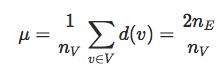The average number of friends that a typical friend has is: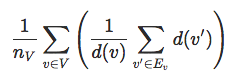But: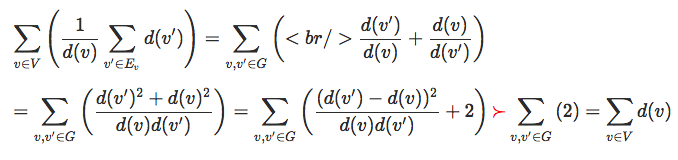Thus: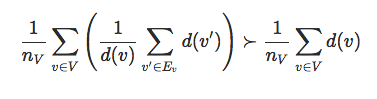Note that this can be related to the variance decomposition: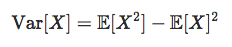i.e.: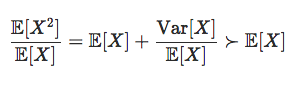(Jensen inequality). But let us get back to our network. The list of nodes is:

M=(rbind(as.matrix(GoT[,1:2]),as.matrix(GoT[,2:1])))
nodes=unique(M[,1])

And we each of them, we can get the list of friends, and the number of friends:

friends = function(x) as.character(M[which(M[,1]==x),2])
nb_friends = Vectorize(function(x) length(friends(x)))

As well as the number of friends our friends have, and the average number of friends.

friends_of_friends = function(y) (Vectorize(function(x) length(friends(x)))(friends(y)))
nb_friends_of_friends = Vectorize(function(x) mean(friends_of_friends(x)))

We can look at the density of the number of friends, for a random node.

Nb  = nb_friends(nodes)
Nb2 = nb_friends_of_friends(nodes)
hist(Nb,breaks=0:40,col=rgb(1,0,0,.2),border="white",probability = TRUE)
lines(density(Nb),col="red",lwd=2)
lines(density(Nb2),col="blue",lwd=2)And we can also compute the averages, just to check:

mean(Nb)
 6.579439
mean(Nb2)
 13.94243

So, indeed, people on average have fewer friends than their friends.

Topics:
big data ,friendship paradox ,data visualization ,statistical analysis

Comment (0)

Save
{{ articles.views | formatCount}} Views

Published at DZone with permission of

Opinions expressed by DZone contributors are their own.

# {{ parent.title || parent.header.title}}

{{ parent.tldr }}

{{ parent.urlSource.name }}